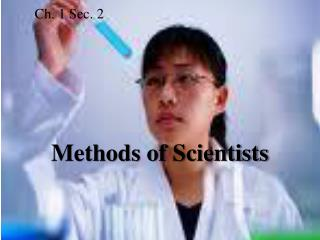DownloadDownload PresentationCh. 1 Sec. 2

# Ch. 1 Sec. 2

Download Presentation## Ch. 1 Sec. 2

- - - - - - - - - - - - - - - - - - - - - - - - - - - E N D - - - - - - - - - - - - - - - - - - - - - - - - - - -
##### Presentation Transcript

1. Ch. 1 Sec. 2 Methods of Scientists

2. 1. Which objects in this photograph are used for measuring? 2. For what kind of measurement is each object used?

3. Review Vocabulary experiment: procedure performed in a controlled setting to test a hypothesis and collect precise data

4. I. The Nature of Scientific Investigation

5. A. Scientific methods - A series of problem solving procedures that help scientists conduct experiments.

6. B. Hypothesis - A testable explanation of a situation that can be supported or disproved by careful procedures

7. C. Experimentation

8. 1. independent variable - the factor that is manipulated by the experimenter a. Only one factor (or variable) should be changed during an experiment

9. 2. dependent variable –factor that is affected by changes in the independent variable

10. 3. control - standard for comparison in an experiment a. Shows results are a condition of variable being tested b. Only one thing changed

11. D. Investigation 1. Observing & Collecting Data 2. Safety

12. E. Analysis & Conclusion Examine results – did they support your hypothesis? Avoid bias or prejudice – do not influence in a particular, typically unfair, direction

13. II. Measurement: Le Système International d’Unités (SI) is a modern version of the metric system based on a decimal system

14. A.Length - 1. The SI unit for length is the meter (m). 2. 1 meter = 39.3700787 inches

15. B. Mass 1. The amount of matter in an object is called mass. 2. The SI unit of mass is the kilogram (kg). 3. 1 kilogram = 2.20462262 pounds

16. C. Weight 1. Weight is a measure of the gravitational force on an object. 2. The SI unit for force is the newton (N). 3. 1 newton = 0.224808943 pounds force

17. D. Area 1. Area is the amount of surface included within a set of boundaries. 2. The SI unit for area is square meters (m2).

18. E. Volume 1. Volume is the amount of space occupied by an object. 2. The SI unit for volume is cubic meters (m3).

19. F. Density 1. Density is the measure of the amount of matter that occupies a given space. 2. The SI units for density areexpressed in grams per cubic centimeter (g/cm3), grams per milliliter (g/mL), and kilograms per cubic meter (kg/m3).

20. G. Time 1. Time is the interval between two events. 2. The SI unit for time is the second (s).

21. 1. Temperature is a measure of the average kinetic energy of the particles that make up a material. 2. The SI unit for temperature is the kelvin (K). 3. Absolute zero is the coldest possible temperature. H. Temperature

22. III. Scientific Notation In scientific notation, a number is expressed as a value between 1 and 10 multiplied by a power of 10.

23. Example The number of grains of sand on Earth is approximately 4,000,000,000,000,000,000,000 and would be written as 4  1021.

24. Example The mass of Earth at 5,974,200,000,000,000,000,000,000 kg would be written as 5.9742  1024 kg.

25. 1 The Nature of Science Why is scientific notation sometimes used when doing science? Answer: It is sometimes necessary when doing science to use numbers that are very large or very small. These numbers can be conveniently expressed by using scientific notation.

26. 1 The Nature of Science Section 1.2 Vocabulary scientific methods hypothesis independent variable dependent variable control Le Système International d’Unités (SI) scientific notation